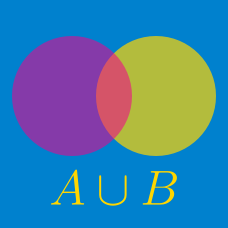Probability

# Union and Intersection

Given the sets $A = \{2, 20, 22, 5, 13\}$ and $B = \{6, 9, 20, 12, 22\}$, what is the sum of all the elements in $A \cup B$?

You may first choose to read the summary page Set Notation.

Given the sets $A = \{17, 29, 65, 1, 19\}$ and $B = \{3, 9, 29, 101, 65\}$, what is the sum of all the elements in $A \cap B$?

Details and assumptions

You may first choose to read the summary page on Set Notation.

A survey of $80$ students found that $42$ of them are wearing red and $34$ of them are wearing green. Furthermore, $27$ of them are wearing neither red nor green. What is the number of students who are wearing red, green, or red and green?

If \begin{aligned} A &= \{x \mid x \text{ is any positive factor of } 52\} \\ B &= \{x \mid x \text{ is any positive factor of } 338\}, \end{aligned}

what is $A \cap B?$

Given the sets $A=\{1,2,3,a,b\}, B=\{3,15,21\},$ if $A \cup B=\{1,2,3,6,10,15,21\},$ what is the value of $a+b?$

×